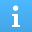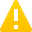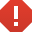# msgbox

## 语法

f = msgbox(message)
f = msgbox(message,title)
f = msgbox(message,title,icon)
f = msgbox(message,title,'custom',icondata,iconcmap)
f = msgbox(___,createmode)

## 说明

f = msgbox(message) 创建一个消息对话框，该对话框可将 message 自动换行，以适应合适大小的图窗。如果您要在多个 App 窗口、MATLAB® 桌面或 Simulink® 上显示消息对话框，并且在响应对话框之前仍能与它们进行交互，请使用此函数。

f = msgbox(message,title) 指定消息框的标题。

f = msgbox(message,title,icon) 指定要在消息对话框中显示的预定义图标。

f = msgbox(message,title,'custom',icondata,iconcmap) 指定要包括在消息对话框中的一个自定义图标。icondata 是定义该图标的图像数据。iconcmap 是用于该图像的颜色图。如果 icondata 是真彩色图像数组，则不需要指定 iconcmap。

f = msgbox(___,createmode) 指定对话框的窗口模式。您也可以指定一个结构体数组，该数组指定窗口模式以及 message 的解释器。

## 示例

f = msgbox('Operation Completed');f = msgbox({'Operation';'Completed'});f = msgbox('Operation Completed','Success');f = msgbox('Invalid Value', 'Error','error');myicon = imread('landOcean.jpg'); h=msgbox('Operation Completed','Success','custom',myicon);[icondata,iconcmap] = imread('trees.tif'); 

h=msgbox('Operation Completed',... 'Success','custom',icondata,iconcmap);h=msgbox('Operation Completed','Success','custom',... icondata,summer);uiwait(msgbox('Operation Completed','Success','modal'));CreateStruct.Interpreter = 'tex'; CreateStruct.WindowStyle = 'modal'; 

h=msgbox('Z = X^2 + Y^2','Value',CreateStruct);## 输入参数

'help''warn''error''none'不显示任何图标。

• 如果 createmode'non-modal'，MATLAB 会使用指定参数创建一个新的非模态消息框。具有相同 title 的现有消息框将继续保留。

• 如果 createmode'modal'，MATLAB 会用指定的模态对话框替换掉最近创建的或点击过的、具有指定 title 的现有消息框。MATLAB 删除其他所有具有相同标题的消息框。被替代的消息框可以是模态消息框，也可以是非模态消息框。

• 如果 createmode'replace'，MATLAB 会用指定的非模态消息框替换掉最近创建的或点击过的、具有指定 title 的消息框。MATLAB 删除其他所有具有相同标题的消息框。被替代的消息框可以是模态消息框，也可以是非模态消息框。

• 如果 createmode 是结构体数组，则它必须具有 WindowStyleInterpreter 字段。WindowStyle 字段的值必须为 'non-modal''modal''replace'Interpreter 字段的值必须为 'tex''none'。如果 Interpreter 值是 'tex'，MATLAB 会将 message 值解释为 TeX。Interpreter 的默认值是 'none'

#### TeX 标记

^{ }上标'text^{superscript}'
_{ }下标'text_{subscript}'
\bf粗体'\bf text'
\it斜体'\it text'
\sl伪斜体（通常与斜体相同）'\sl text'
\rm常规字体'\rm text'
\fontname{specifier}字体名称 - 将 specifier 替换为字体系列的名称。您可以将此说明符与其他修饰符结合使用。'\fontname{Courier} text'
\fontsize{specifier}字体大小 - 将 specifier 替换为以磅为单位的数值标量值。'\fontsize{15} text'
\color{specifier}字体颜色 - 将 specifier 替换为以下颜色之一：redgreenyellowmagentablueblackwhitegraydarkGreenorangelightBlue'\color{magenta} text'
\color[rgb]{specifier}自定义字体颜色 - 将 specifier 替换为三元素 RGB 三元组。'\color[rgb]{0,0.5,0.5} text'

\alpha

α

\upsilon

υ

\sim

~

\angle

\phi\leq

\ast

*

\chi

χ

\infty

\beta

β

\psi

ψ

\clubsuit

\gamma

γ

\omega

ω

\diamondsuit

\delta

δ

\Gamma

Γ

\heartsuit

\epsilon

ϵ

\Delta

Δ

\spadesuit

\zeta

ζ

\Theta

Θ

\leftrightarrow

\eta

η

\Lambda

Λ

\leftarrow

\theta

θ

\Xi

Ξ

\Leftarrow

\vartheta

ϑ

\Pi

Π

\uparrow

\iota

ι

\Sigma

Σ

\rightarrow

\kappa

κ

\Upsilon

ϒ

\Rightarrow

\lambda

λ

\Phi

Φ

\downarrow

\mu

µ

\Psi

Ψ

\circ

º

\nu

ν

\Omega

Ω

\pm

±

\xi

ξ

\forall

\geq

\pi

π

\exists

\propto

\rho

ρ

\ni

\partial

\sigma

σ

\cong

\bullet

\varsigma

ς

\approx

\div

÷

\tau

τ

\Re

\neq

\equiv

\oplus

\aleph

\Im

\cup

\wp

\otimes

\subseteq

\oslash

\cap

\in

\supseteq

\supset

\lceil

\subset

\int

\cdot

·

\o

ο

\rfloor

\neg

¬

\nabla

\lfloor

\times

x

\ldots

...

\perp

\surd

\prime

´

\wedge

\varpi

ϖ

\0

\rceil

\rangle

\mid

|

\vee

\langle

\copyright